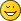Acceleration spectrum - Printable Version +- phyphox Forums (https://phyphox.org/forums) +-- Forum: Discussion (https://phyphox.org/forums/forumdisplay.php?fid=1) +--- Forum: Experiments and Ideas (https://phyphox.org/forums/forumdisplay.php?fid=4) +--- Thread: Acceleration spectrum (/showthread.php?tid=1661) Acceleration spectrum - Rambo32 - 12-26-2022 Hi to everyone, I'm an Engineering student from Italy. I'm trying to measure aceleration spectrum during a path, but I obtained different results between the Spectrum from Phyphox e the spectrum obrained from raw data using fft on Matlab. I used experiment acceleration spectrum, and even considering a correction factor in the two spectra they are quite different. To be more clear I attach some graphs. Can you help me to understand what's happening? Whatt calculation phyphox does for the fft? Thanks in advance. RE: Acceleration spectrum - solid - 12-27-2022 Hello. Interesting observation. For a comparison of two fft it would be useful to see you matlab program. With brest wishes. Mikhail. RE: Acceleration spectrum - Rambo32 - 12-27-2022 (12-27-2022, 06:07 PM)solid Wrote: Hello. Interesting observation. For a comparison of two fft it would be useful to see you matlab program. With brest wishes. Mikhail. Thanks for your interest. I attach the matlab code screen, if needed I can provide the whole script/collected data. RE: Acceleration spectrum - solid - 12-27-2022 Hi. You first calculate some kind of acceleration amlitude and then apply fft. As I remember (to verify) phyphox first apply fft to every component and then calculate an amplitude. It's not the same... RE: Acceleration spectrum - Rambo32 - 12-29-2022 (12-27-2022, 11:43 PM)solid Wrote: Hi. You first calculate some kind of acceleration amlitude and then apply fft. As I remember (to verify) phyphox first apply fft to every component and then calculate an amplitude. It's not the same... I just tried in this way: results are still slightly different but much better! Thanks. RE: Acceleration spectrum - Jens Noritzsch - 12-29-2022 Mikhail is right on his assumption, see https://github.com/phyphox/phyphox-experiments/blob/master/acc_spectrum.phyphoxRoughly summarized: phyphox computes the complex fft for each accelerometer component data, then it forms for each the complex absolute value squared, adds these three, and takes the square root. BTW, subtracting 9.81 does not appear to make sense: this is not how vector addition is supposed to work…